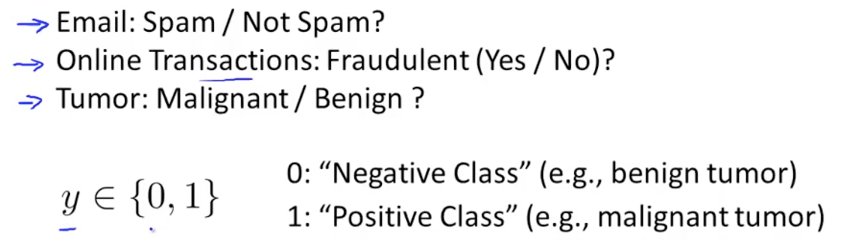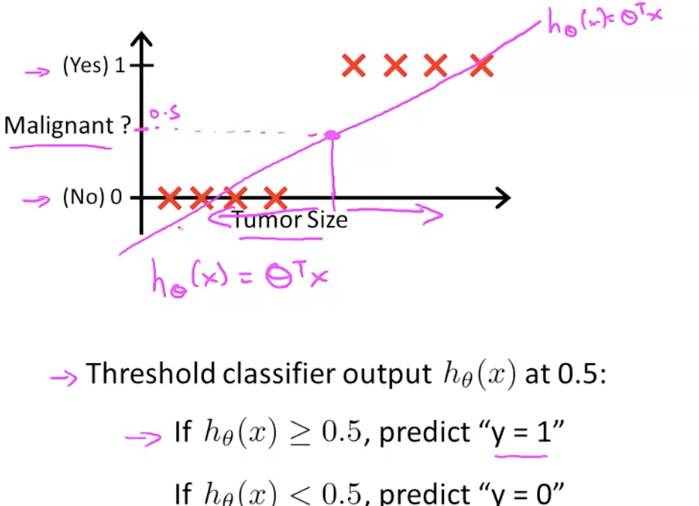# Machine Learning學習日記 — Coursera篇 (Week 3.1):Logistic Regression, Classification, Hypothesis Representation, Decision Boundary

### 大綱

1. Classification
2. Hypothesis Representation
3. Decision Boundary

### 1. ClassificationTransactions：交易/ Fraudulent：欺詐的/ Tumor：腫瘤/ Malignant：惡性的/ Benign：良性的Threshold：門檻/ classifier：分類器

Linear Regression解決不了classification的問題

Logistic “Regression”只是慣用詞，與Regression本身沒什麼太大關係

Ans:

### 2. Hypothesis Representation

The function that represents the "Logistic Regression"

Logistic regression引進了sigmoid函式的概念

g(z)即sigmoid function，而z為θ的轉置矩陣乘以x。

Ans：

### 3. Decision Boundary

z就是θ的轉置矩陣乘上x

(把z展開，同時給予θi(i=0-2)的值)

#### 隨堂練習

Ans：

Step 1：求出decision boundary的函式
z = 5 - x1，當z>0的時候代表預測為惡性腫瘤(y=1)

Step 2：繪出圖形，基本上有兩種可能
Step 3：判斷哪邊為惡性(y=1)，哪邊為良性(y=0)